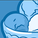# QlikView App Dev

Discussion Board for collaboration related to QlikView App Development.

Announcements
On May 18th at 10AM EDT we will answer your QlikView questions live. REGISTER
cancel
Showing results for
Did you mean:Contributor II

## Sum Aggr and Count with Multiple If Else Conditions

Good Day All,

I am working on store related data and i have to calculate compliance on each visit for a KPI but the problem is we have different store types with different criteria applied on it.

I have write below expression but there is some mistake in it, can you please help me out where i am making the mistake.

Expression:

sum(aggr(
If(count
(distinct
if
(Parameter='KPI-1' and Compliance='C' and Store_Type<>'Cosmetics',Category)
)>=5,1,if(Parameter='KPI-1' and Compliance='C' and Store_Type='Cosmetics',Category)>=2,1)
,Visit,Compliance))

Criteria:

Grocers =5, Cosmetics=2Labels (3)

• ### If Else Expression

1 Solution

Accepted SolutionsMVP

You can try below

``````sum(aggr(if(Count({<Parameter={'KPI-1'},Compliance={'C'},Store_Type={'Grocers'}>}Visit)>=5,1),Store_Type,Compliance,Visit))+
sum(aggr(if(Count({<Parameter={'KPI-1'},Compliance={'C'},Store_Type={'Cosmetics'}>}Visit)>=2,1),Store_Type,Compliance,Visit))``````
3 RepliesMVP

How you are getting total visit as 4?Contributor II
Author

For Total Visits:

=Sum( Aggr(Count(DISTINCT if(Parameter='KPI-1', Visit)), Storecode))MVP

You can try below

``````sum(aggr(if(Count({<Parameter={'KPI-1'},Compliance={'C'},Store_Type={'Grocers'}>}Visit)>=5,1),Store_Type,Compliance,Visit))+
sum(aggr(if(Count({<Parameter={'KPI-1'},Compliance={'C'},Store_Type={'Cosmetics'}>}Visit)>=2,1),Store_Type,Compliance,Visit))``````